# 【深度学习】深入浅出CRF as RNN（以RNN形式做CRF后处理）1人已赏
Fizz 发布于 2021-06-09 17:29:12 浏览 2536 点赞 93 收藏 5

## 【深度学习】深入浅出CRF as RNN（以RNN形式做CRF后处理）

``````文章目录
1 概述
2 目标
3 思路
4 简述
5 论文原文
5.1 Introduction
5.2 相关工作
5.3 关键步骤
6 仓库代码``````

# 1 概述

1.1 马尔科夫链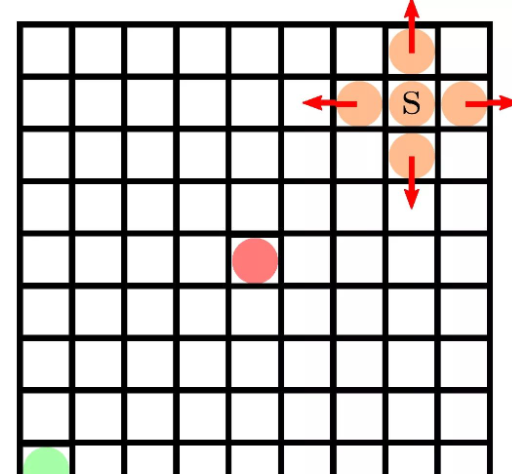1.2 隐式马尔科夫模型

CRF是一种判别式概率模型，是随机场的一种，结合了最大熵模型和隐式马尔科夫模型的特点；CRF是一种无向图模型，图中的顶点代表随机变量，顶点间的连线代表随机变量间的相依关系。其条件概率分布模型可以表述为P(Y|X)，即给定一组随机变量的条件下，随机变量Y的马尔科夫随机场（MRF，Markov Random Field）。

# 2 目标

{1,2,⋯,N}{1,2,⋯,N}

# 4 简述

1. 用训练的方式求解CRF。
2. 把CRF挂在一个FCN后边，一起训练。
3. 求解CRF时，用独立分布近似真实分布，其算法可以包装成一个RNN。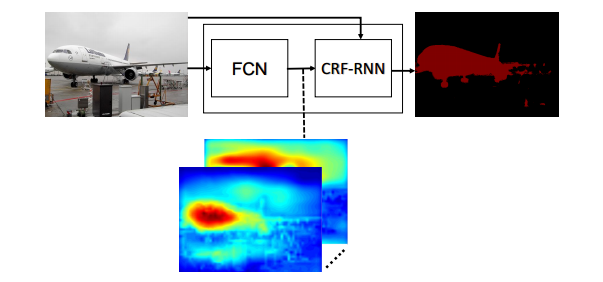# 5 论文原文

## 5.1 Introduction

FCN开辟了卷积网络用于像素级语义分割的新思路，为CNN与CRF结合提供了必要的技术基础。但其输出的分割结果比较边界粗糙。

DeepLab使用CRF来改进FCN的结果。但是CRF只作为一个后处理工具，不参与训练，所以不能发挥出CRF的全部威力。

## 5.3 关键步骤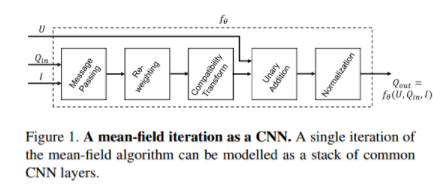将上述堆叠的Layers作为一个大模块，挂载到一个RNN网络结构中。RNN的网络结构如图所示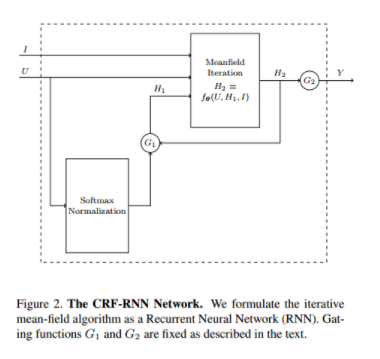求解CRF的这个RNN网络，又作为整体网络的一部分，挂在FCN网络之后。

# 6 仓库代码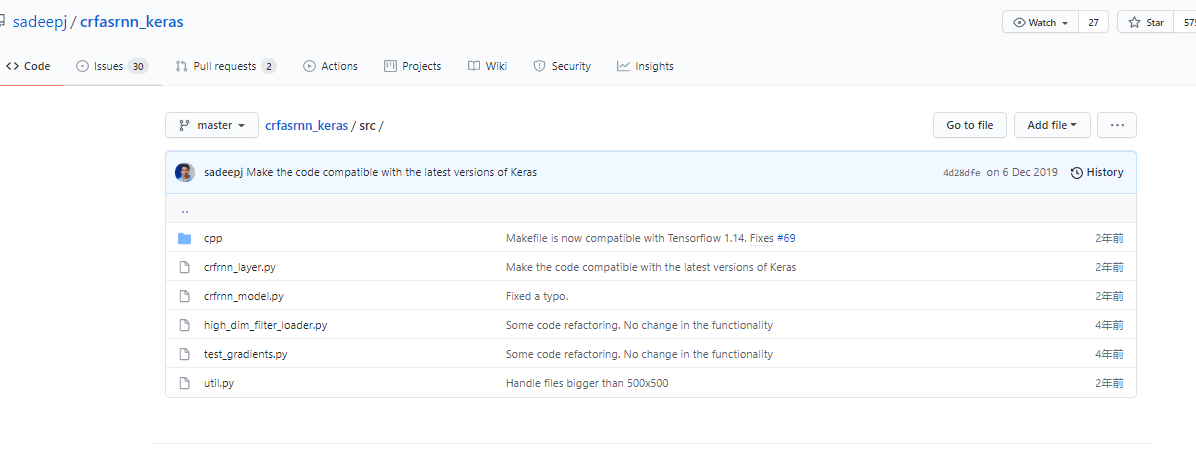``````class CrfRnnLayer(Layer):
""" Implements the CRF-RNN layer described in:
Conditional Random Fields as Recurrent Neural Networks,
S. Zheng, S. Jayasumana, B. Romera-Paredes, V. Vineet, Z. Su, D. Du, C. Huang and P. Torr,
ICCV 2015
"""

def __init__(self, image_dims, num_classes,
theta_alpha, theta_beta, theta_gamma,
num_iterations, **kwargs):
self.image_dims = image_dims
self.num_classes = num_classes
self.theta_alpha = theta_alpha
self.theta_beta = theta_beta
self.theta_gamma = theta_gamma
self.num_iterations = num_iterations
self.spatial_ker_weights = None
self.bilateral_ker_weights = None
self.compatibility_matrix = None
super(CrfRnnLayer, self).__init__(**kwargs)

def build(self, input_shape):
# Weights of the spatial kernel
self.spatial_ker_weights = self.add_weight(name='spatial_ker_weights',
shape=(self.num_classes, self.num_classes),
initializer=_diagonal_initializer,
trainable=True)

# Weights of the bilateral kernel
self.bilateral_ker_weights = self.add_weight(name='bilateral_ker_weights',
shape=(self.num_classes, self.num_classes),
initializer=_diagonal_initializer,
trainable=True)

# Compatibility matrix
self.compatibility_matrix = self.add_weight(name='compatibility_matrix',
shape=(self.num_classes, self.num_classes),
initializer=_potts_model_initializer,
trainable=True)

super(CrfRnnLayer, self).build(input_shape)

def call(self, inputs):
unaries = tf.transpose(inputs[0, :, :, :], perm=(2, 0, 1))
rgb = tf.transpose(inputs[0, :, :, :], perm=(2, 0, 1))

c, h, w = self.num_classes, self.image_dims, self.image_dims
all_ones = np.ones((c, h, w), dtype=np.float32)

# Prepare filter normalization coefficients
spatial_norm_vals = custom_module.high_dim_filter(all_ones, rgb, bilateral=False,
theta_gamma=self.theta_gamma)
bilateral_norm_vals = custom_module.high_dim_filter(all_ones, rgb, bilateral=True,
theta_alpha=self.theta_alpha,
theta_beta=self.theta_beta)
q_values = unaries

for i in range(self.num_iterations):
softmax_out = tf.nn.softmax(q_values, 0)

# Spatial filtering
spatial_out = custom_module.high_dim_filter(softmax_out, rgb, bilateral=False,
theta_gamma=self.theta_gamma)
spatial_out = spatial_out / spatial_norm_vals

# Bilateral filtering
bilateral_out = custom_module.high_dim_filter(softmax_out, rgb, bilateral=True,
theta_alpha=self.theta_alpha,
theta_beta=self.theta_beta)
bilateral_out = bilateral_out / bilateral_norm_vals

# Weighting filter outputs
message_passing = (tf.matmul(self.spatial_ker_weights,
tf.reshape(spatial_out, (c, -1))) +
tf.matmul(self.bilateral_ker_weights,
tf.reshape(bilateral_out, (c, -1))))

# Compatibility transform
pairwise = tf.matmul(self.compatibility_matrix, message_passing)

# Adding unary potentials
pairwise = tf.reshape(pairwise, (c, h, w))
q_values = unaries - pairwise

return tf.transpose(tf.reshape(q_values, (1, c, h, w)), perm=(0, 2, 3, 1))

def compute_output_shape(self, input_shape):
return input_shape``````

``\$ git clone https://github.com/sadeepj/crfasrnn_keras.git``

``````\$ cd crfasrnn_keras
\$ pip install -r requirements.txt  # If you have a GPU device, use requirements_gpu.txt instead``````

``````\$ python
import tensorflow
mport keras``````

``````\$ cd crfasrnn_keras/src/cpp
\$ make``````

``````\$ cd crfasrnn_keras
\$ python run_demo.py``````

*本文仅代表作者观点，不代表易百纳技术社区立场。系作者授权易百纳技术社区发表，未经许可不得转载。

### 相关专栏海思讨论群君正智能视频群客服微信： x460910098客服手机： 17714209983